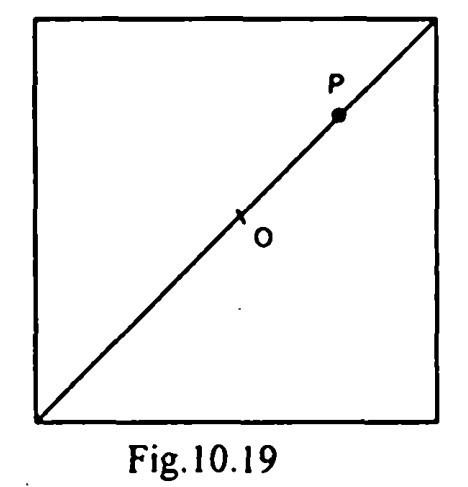Search

# Strength of Materials(161-180)

Updated: Apr 28, 2020

161. A cantilever beam of uniform EI has span equal to 'L' . An upward force W acts at the mid point of the beam and a downward force P acts at the free end. In

order that the deflection at the free end is zero, the relation between P and W should be W = 16P/5

162. A wooden beam of width B and depth D is strengthened by two steel plates of thickness 't' and depth 'D' on both sides of the beam. The allowable bending stress in wood is a and modular ratio of steel to wood is 'm'. The allowable bending

moment is163. If K is defined as the ratio of Young's modulus of elasticity and the permissible

stress in compression of a material used in the construction of a column, then the

Rankine's constant used in finding the load carrying capacity of columns is

proportional to 1/K

164. A load P is acting on the diagonal of a square column of size 'D' as shown in

Fig. For no tension, the maximum distance of the load from the centre 'O' isD/(2/6)

165. The force in member ® of the truss shown in Fig. is16T tensile

166. A circular shaft subjected to torsion undergoes a twist of 1° in a length of 120

cm. If the maximum shear stress induced is limited to 1000 kg/cm² and if modulus of rigidity G = 0.8 x 106 kg/cm², then the radius of the shaft should be 27/π

167. A three hinged parabolic arch rib with hinges at abutments and at crown is under the action of uniformly distributed load ' W" per unit length over its entire span 'L' through its crown. The bending moment at quarter span is zero

168. In order to produce bending and shear stresses of equal magnitudes at the

extreme fibres of a circular cross section under the action of combined bending and torsion, the ratio of bending to twisting moments must be 1/2

169.170. A bar AB, 4 cm in diameter and 4 m long is rigidly fixed at its ends. A torque of

12,000 kg. cm is applied at a section of the bar, one metre from end A. The fixing

couples TA and TB (in kg. cm) at the supports A and B will be respectively,

9000,3000

171. Given that the density of earth is w, the angle of repose is Φ and h is the height of the wall and the earth retrained is level, the active earth pressure 'P' on a retaining wall is given by172. Creep is time dependent deformation under stress

173. In a close coiled helical spring subjected to an axial load, other quantities remaining the same , if the wire diameter is doubled, then the stiffness of the spring when compared to the original one, will become sixteen times

174. A compound bar consisting of material A and B is tightly secured at the ends. The coefficient of thermal expansion of A is more than that of B. When the

temperature is increased the stresses induced will be compressive in material A and tensile in material B

175. A single direct stress 'p' acts along the longitudinal axis (x-axis) of a bar of

rectangular cross section. E is the Young's modulus and 1/m is the Poisson's ratio of the material. Match list I with List II and select the correct answer using the codes given below the lists

176.177.

1.Welded structures are usually lighter than riveted structures. 2.Welding allows the arrangement of structural components in such a manner that the joint provides maximum efficiency

178.The shear stress distribution over a rectangular cross section of a beam follows

In -the Fig. 10.21, 0\ and a2 are the maximum and minimum principal

stresses. In order that the resultant stress on the plane AB isthe value of θ should be

45°

179.Four vertical columns of the same material, height and weight have the same

end conditions. The buckling load will be the largest for a column having the cross section of a/an thin hollow cylinder

180. If all the dimensions of a prismatic bar elongating under its own weight are

increased in the proportion m:l, then the total elongation will increase in the ratio1 : m